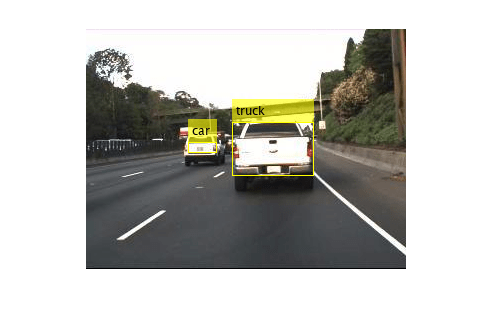predict

Compute YOLO v3 deep learning network outputs for inference

Description

example

output = predict(detector,dlX) computes the YOLO v3 deep learning network outputs during inference, given the detector and the test data. Use this function to get predictions from the output layers of the YOLO v3 deep learning network during inference.

Note

This function requires the Computer Vision Toolbox™ Model for YOLO v3 Object Detection. You can install the Computer Vision Toolbox Model for YOLO v3 Object Detection from Add-On Explorer. For more information about installing add-ons, see Get and Manage Add-Ons. To run this function, you will require the Deep Learning Toolbox™.

Examples

collapse all

Load a pretrained YOLO v3 object detector.

detector = yolov3ObjectDetector('tiny-yolov3-coco');

Load the test image for prediction.

Preprocess the test image and convert the preprocessed image to a formatted dlarray object.

[Ip,info] = preprocess(detector,I);
Ip = im2single(Ip);
dlX = dlarray(Ip,'SSCB');

Compute predictions for the test image. The predict function returns the predictions for the feature maps from the output layers of the YOLO v3 deep learning network. The first column contains the confidence scores. Columns 2 to 5 contain the bounding box locations computed relative to the grid cell coordinates. The sixth column contains the class probabilities for each class used during training. The seventh and the eighth column contains the prior width and prior height of bounding boxes as computed by the network, respectively.

output = predict(detector,dlX)
output=2×8 cell array
{13×13×3×1 dlarray}    {13×13×3×1 dlarray}    {13×13×3×1 dlarray}    {13×13×3×1 dlarray}    {13×13×3×1 dlarray}    {13×13×240×1 dlarray}    {13×13×3×1 dlarray}    {13×13×3×1 dlarray}
{26×26×3×1 dlarray}    {26×26×3×1 dlarray}    {26×26×3×1 dlarray}    {26×26×3×1 dlarray}    {26×26×3×1 dlarray}    {26×26×240×1 dlarray}    {26×26×3×1 dlarray}    {26×26×3×1 dlarray}

You can then get the final detections by using the predictions for features with maximum objectness scores. The objectness score is the product of confidence score and class probability. To compute the exact bounding box location, you must map the predicted bounding box values to box coordinates. Alternatively, you can use the detect function to directly get the detection results. The detect function internally calls the predict function to compute the feature maps.Input Arguments

collapse all

YOLO v3 object detector, specified as a yolov3ObjectDetector object.

Test data, specified as a formatted dlarray (Deep Learning Toolbox) object. The test data can contain one or more test images.

Output Arguments

collapse all

Output predictions, returned as an N-by-8 cell array of formatted dlarray (Deep Learning Toolbox) objects. N is the number of output layers in the YOLO v3 deep learning network. Each row in the cell array is of form [conf bx by bw bh prob tw th]. The function returns predictions as a formatted dlarray (Deep Learning Toolbox) value..

 Predictions Description conf Confidence scores for each bounding box. bx X-coordinate of the center of the predicted bounding box relative to the location of the grid cell. by Y-coordinate of the center of the predicted bounding box relative to the location of the grid cell. bw Width of the predicted bounding box relative to the location of the grid cell. bh Height of the predicted bounding boxes relative to the location of the grid cell. prob Class probabilities predicted for each feature in the output feature map. tw Prior width of the bounding boxes as computed by the network. th Prior height of the bounding boxes as computed by the network.